jph·2021년 8월 9일
0

목록 보기
1/2

# Notation

this section provides a concise reference describing the notation used throughout this book.

if you are unfamiliar with any of the corresponding mathematical concepts, we describe most of these ideas in chapters 2-4.

## Numbers and Arrays

$a$

A scalar (integer or real)

$\textbf{a}$

A Vector

$\textbf{A}$

A matrix

$\Alpha$

A tensor

$I_n$

Identity matrix with n rows and n columns

$e^{(i)}$

Standard basis vector [0, . . . ,0,1,0, . . . ,0] with a 1 at position i

$diag(\textbf{a})$

a square, diagonal matrix with diagonal entries given by 'a'

## Sets and Graphs

$\mathbb{A}$

'A' set

$\mathbb{R}$

The set of real numbers

{0,1}

The set containing 0 and 1

{0,1.....,n}

The set of all integers between 0 and n

$[a,b]$

The real interval including a and b

$(a,b]$

The real interval excluding a but including b

$\mathbb{A}/\mathbb{B}$

Set subtraction, i.e., the set containing the elements of A that are not in B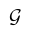A graph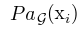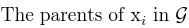## Indexing

$a_i$

Elements i of vector 'a' , with indexing starting at 1

$a_-i$

All elements of vector 'a' except for element i

$A_{ij}$

Elements i,j of matrix A

$A_{i,:}$

Row i of matrix A

$A_{:,i}$

Column i of matrix A

$A_{i,j,k}$

Elements (i,j,k) of a 3-D tensor A

## Linear Algebra operations

$A^T$

Transpose of matrix A

$A^+$

Moore-Penrose pseudoinverse of A

$A \bigodot B$

Element-wise (Hadamard) product of A and B

$det(A)$

Determinant of A

## Calculus

$\frac{dy}{dx}$

Derivative of y with respect to x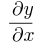Partial derivative of y with respect to x

$\bigtriangledown_xy$

Gradient of y with respect to x

$\bigtriangledown_Xy$

Matrix derivatives of y with respect to X

$\bigtriangledown\textbf{x}y$

Tensor containing derivatives of y with respect to X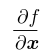Jacobian matrix

$J \in \mathbb{R}^{m * n} of \\ f :\mathbb{R} \rightarrow \mathbb{R}^m$

$\int \mathrm{f}(x)dx$

define integral over the entire domain of x

$\int _\mathbb{S} f(x)dx$

Definite integral with respect to x over the set S

## Probability and Information Theory

$a\perp b$

The random variables a and b are independent

$a\perp b \mid c$

They are conditionally independent given c\

$P(a)$

a probability distribution over a discrete variable

$p(a)$

A probability distribution over a continuous variable, or over a variable whose type has not been specified

$a \sim P$

Random variable a has distribution P

$\mathbb{E}_{x \sim P}[f(x)] or \mathbb{E}f(x)$

Expectation of f(x) with respect to P(x)

$Var(f(x))$

Variance of f(x) under P(x)

$Cov(f(x),g(x))$

Covariance of f(x) and g(x) under P(x)

$H(x)$

Shannon entropy of the random variable x

$D_{KL}(P)\parallel Q$

Kullback-Leibler divergence of P and Q

$\mathcal{N} (x;u,\sum)$

Gaussian distribution over x with mean µ and covariance Σ

## Function

$f:\mathbb{A} \to \mathbb{B}$

The function f with domain A and range B

$f \circ g$

Composition of the functions f and g

$f(x;\theta)$

A function of x parametrized by theta, (Sometimes we write f(x) and omit the argument theta to lighten notation)

$logx$

Natural logarithm of x

$\alpha(x)$

Logistic sigmoid,

$\frac{1}{1+exp(-x)}$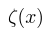Softplus, log(1_exp(x))

$\parallel x \parallel_{p}$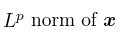$\parallel x \parallel$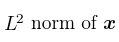$x^+$

Positive part of x , i.e., max(0,x)

$1_{condition}$

is 1 if the condition is true, 0 otherwise

## Datasets and Distributions

$P_{data}$

The data generating distribution

$\hat{P}_{data}$

The empirical distribution defined by the training set

$\mathbb{X}$

A set of training examples

$x^{i}$

The i-th example (input) from a dataset

$y^{i} or y^{i}$

The target associated with x^{i} for supervised learning

$X$

The m x n matrix with input example x^{i} in row Xi: